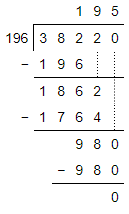Home/Class 10 Math Chapter List/1. Real Numbers/

# Use Euclid’s division algorithm to find the HCF of (196) and (38220).

## QuestionMathClass 10

Use Euclid’s division algorithm to find the HCF of $$196$$ and $$38220$$.

$$196$$
4.64.6## Solution

We have,Dividend $$=38220$$ and Divisor $$=196$$
By Euclid's Division Lemma
Dividend $$=$$ $$($$Divisor $$\times$$ Quotient $$+$$ Remainder$$)$$Therefore, $$38220=196\times195+0$$
As remainder $$= 0$$ when divisor$$=196$$ therefore by Euclid's Division Algorithm, when remainder becomes $$0$$ , divisor is the $$HCF$$
Hence, HCF $$(196,38220)=196$$.